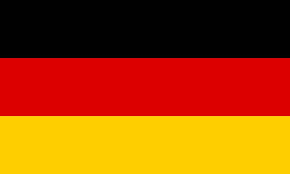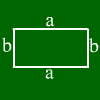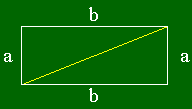# Rectangle calculationEnter two values. Other values will be calculated.Side a: Side b: area Perimeter: diagonal
Enter for points in the plane. Mathepower calculates what quadrangle they form.
A(|)

B(|)

C(|)

D(|)

Enter the coordinates of for points. Mathepower calculates what (if any) quadrangle they form
A(||)

B(||)

C(||)

D(||)

For a rectangle with sides a and b, the following formulas hold:
Area = a*b
Perimeter = 2*a+2*b
Diagonal = square root of a˛+b˛

## What is a rectangle?Here we can see a rectangle.
A rectangle has those typical properties:
It's got four sides and four corners.
The sides are perpendicular to each other, i.e. they inclose a 90°-angle.
Two opposing sides are parallel.
Opposing sides have the same length.

## How to do rectangle calculations?

The interior area can be calculated as a*b where a is one side and b the other one.
The yellow line (called diagonal) has the length square root of (a^2+b^2).
If you want to see some examples, just enter your example above.

For further information, just move the mouse over one of the words above. The corresponding piece of the rectangle will then be marked.Side a Side b
Diagonal d
Area
Perimeter

## Rectangle calculation

Mathepower calculates perimeter, area, side and diagonal of a rectangle. You just have to enter e.g. both side lengths or any other two values.